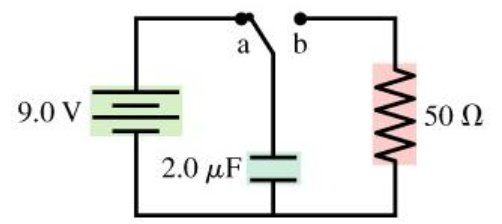# Problem: The switch in the figure has been in position a for a long time. It is changed to position b at t = 0 s.A. What is the charge Q on the capacitor immediately after the switch is closed?B. What is the current I through the resistors immediately after the switch is closed?

###### FREE Expert Solution

Charge on a capacitor is expressed as Q = CV

Expression of voltage across the capacitor is:

$\overline{){{\mathbf{V}}}_{{\mathbf{C}}}{\mathbf{\left(}}{\mathbf{t}}{\mathbf{\right)}}{\mathbf{=}}{{\mathbf{V}}}_{{\mathbf{0}}}{{\mathbf{e}}}^{\frac{\mathbf{-}\mathbf{t}}{\mathbf{\tau }}}}$

or

$\overline{){{\mathbf{V}}}_{{\mathbf{C}}}{\mathbf{\left(}}{\mathbf{t}}{\mathbf{\right)}}{\mathbf{=}}{{\mathbf{V}}}_{{\mathbf{0}}}{{\mathbf{e}}}^{\frac{\mathbf{-}\mathbf{t}}{\mathbf{R}\mathbf{C}}}}$

Since the time constant τ is given as, τ = RC

84% (339 ratings)###### Problem DetailsThe switch in the figure has been in position a for a long time. It is changed to position b at t = 0 s.

A. What is the charge Q on the capacitor immediately after the switch is closed?

B. What is the current I through the resistors immediately after the switch is closed?